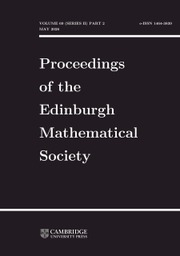Home
Hostname: page-component-79b67bcb76-wlt4x Total loading time: 0.219 Render date: 2021-05-14T20:59:09.607Z Has data issue: true Feature Flags: { "shouldUseShareProductTool": true, "shouldUseHypothesis": true, "isUnsiloEnabled": true, "metricsAbstractViews": false, "figures": false, "newCiteModal": false, "newCitedByModal": true, "newEcommerce": true }Proceedings of the Edinburgh Mathematical Society

# COUNTABLE VERSUS UNCOUNTABLE RANKS IN INFINITE SEMIGROUPS OF TRANSFORMATIONS AND RELATIONS

Published online by Cambridge University Press:  10 December 2003

Corresponding

## Abstract

The relative rank $\rank(S:A)$ of a subset $A$ of a semigroup $S$ is the minimum cardinality of a set $B$ such that $\langle A\cup B\rangle=S$. It follows from a result of Sierpiński that, if $X$ is infinite, the relative rank of a subset of the full transformation semigroup $\mathcal{T}_{X}$ is either uncountable or at most $2$. A similar result holds for the semigroup $\mathcal{B}_{X}$ of binary relations on $X$.

A subset $S$ of $\mathcal{T}_{\mathbb{N}}$ is dominated (by $U$) if there exists a countable subset $U$ of $\mathcal{T}_{\mathbb{N}}$ with the property that for each $\sigma$ in $S$ there exists $\mu$ in $U$ such that $i\sigma\le i\mu$ for all $i$ in $\mathbb{N}$. It is shown that every dominated subset of $\mathcal{T}_{\mathbb{N}}$ is of uncountable relative rank. As a consequence, the monoid of all contractions in $\mathcal{T}_{\mathbb{N}}$ (mappings $\alpha$ with the property that $|i\alpha-j\alpha|\le|i-j|$ for all $i$ and $j$) is of uncountable relative rank.

It is shown (among other results) that $\rank(\mathcal{B}_{X}:\mathcal{T}_{X})=1$ and that $\rank(\mathcal{B}_{X}:\mathcal{I}_{X})=1$ (where $\mathcal{I}_{X}$ is the symmetric inverse semigroup on $X$). By contrast, if $\mathcal{S}_{X}$ is the symmetric group, $\rank(\mathcal{B}_{X}:\mathcal{S}_{X})=2$.

AMS 2000 Mathematics subject classification: Primary 20M20

## Keywords

Type
Research Article
InformationYou have Access

# Send article to Kindle

Note you can select to send to either the @free.kindle.com or @kindle.com variations. ‘@free.kindle.com’ emails are free but can only be sent to your device when it is connected to wi-fi. ‘@kindle.com’ emails can be delivered even when you are not connected to wi-fi, but note that service fees apply.

Find out more about the Kindle Personal Document Service.

COUNTABLE VERSUS UNCOUNTABLE RANKS IN INFINITE SEMIGROUPS OF TRANSFORMATIONS AND RELATIONS
Available formats
×

# Send article to Dropbox

To send this article to your Dropbox account, please select one or more formats and confirm that you agree to abide by our usage policies. If this is the first time you use this feature, you will be asked to authorise Cambridge Core to connect with your <service> account. Find out more about sending content to Dropbox.

COUNTABLE VERSUS UNCOUNTABLE RANKS IN INFINITE SEMIGROUPS OF TRANSFORMATIONS AND RELATIONS
Available formats
×

# Send article to Google Drive

To send this article to your Google Drive account, please select one or more formats and confirm that you agree to abide by our usage policies. If this is the first time you use this feature, you will be asked to authorise Cambridge Core to connect with your <service> account. Find out more about sending content to Google Drive.

COUNTABLE VERSUS UNCOUNTABLE RANKS IN INFINITE SEMIGROUPS OF TRANSFORMATIONS AND RELATIONS
Available formats
×
×